Maths
Study Material

# Rectangular Hyperbola

As we know that an ellipse is a special type of circle; likewise rectangular hyperbola is a special type of hyperbola.

7 minutes long
Posted by Kunduz Tutor, 18/11/2021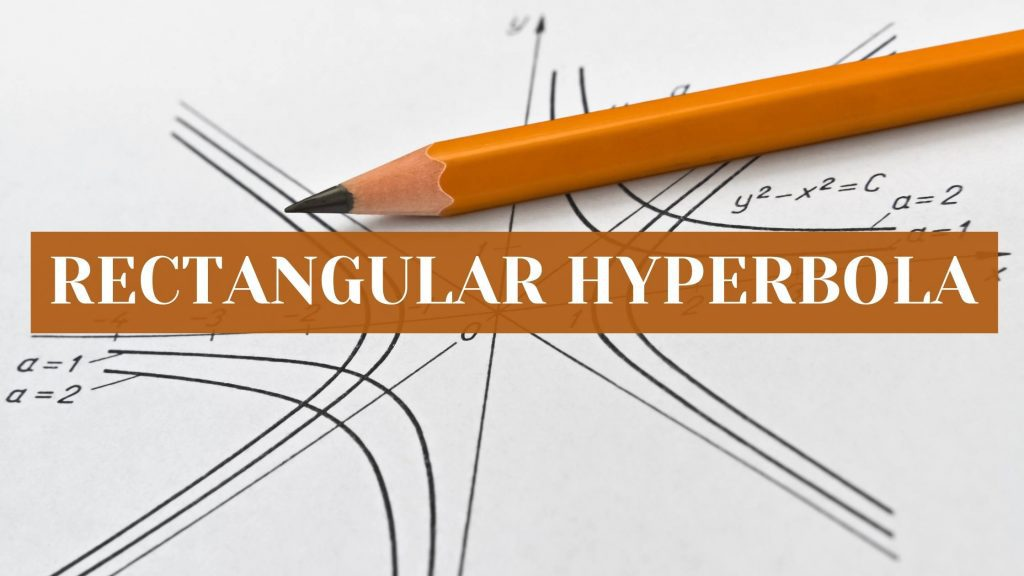Hesap Oluştur

Got stuck on homework? Get your step-by-step solutions from real tutors in minutes! 24/7. Unlimited.

As we know that an ellipse is a special type of circle; likewise rectangular hyperbola is a special type of hyperbola. There are a few special types of hyperbola(s), out of which one is the rectangular hyperbola. To define a rect. hyperbola, the number of equivalent ways is more than one.

Author – Ojasvi Chaplot

## Rectangular Hyperbola Definition

A rectangular hyperbola can be described as the hyperbola whose asymptotes are at right angles to each other.

## Rectangular Hyperbola Equation

The angle between the asymptotes of the hyperbola: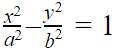,  is given as: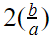So, this is a right-angle,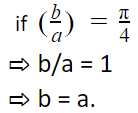So, both the axes, the transverse axis and the conjugate axis are equal. Therefore, the equation of rect. hyperbola is written as: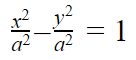Hence, the equation of rectangular hyperbola is: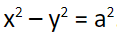## Eccentricity of Rectangular Hyperbola

Since, the eccentricity of the hyperbola is obtained by: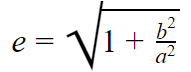Then, the eccentricity of rectangular hyperbola is: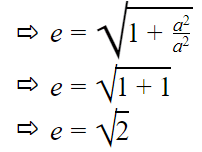Rect. Hyperbola is a special type of hyperbola in the conic section, the eccentricity of which is equal to √2. Rect. hyperbola is also referred to as equilateral hyperbola.

Note – It can also be called as square hyperbola. Let us explain why we can call it square. When the asymptotes of the hyperbola are drawn and then a rectangle is drawn with the help of those asymptotes, so we will observe that the length is equal to the breadth of that rectangle in a few cases.

## Important Points of Rectangular Hyperbola

• In a hyperbola, since b2 = a2 (e2 – 1). In the case of rect. hyperbola (i.e., when b = a), it becomes
• a2 = a2(e2 – 1)
• e2 = 2
• e = √2
• i.e. the eccentricity of a rect. hyperbola is equal to √2.
• In case of the rect. hyperbola, since a = b, therefore the length of the transverse axis is equal to the length of the conjugate axis.
• A rect. hyperbola is also referred as an equilateral hyperbola.
• The asymptotes of the rect. hyperbola are y = ± x.

### Special Case

If the axes of the hyperbola are rotated by an angle of (-π/4) about the same origin, then the equation of the rect. hyperbola x2 – y2 = ais reduced to xy = c2; where c2 = a2/2, which can be illustrated as shown:

So, for this we have to write [(x/√2 + y/√2) for x] and [(–x/√2 + y/√2) for y].

Then, the equation becomes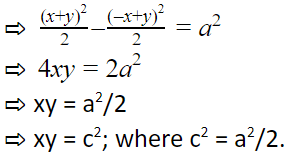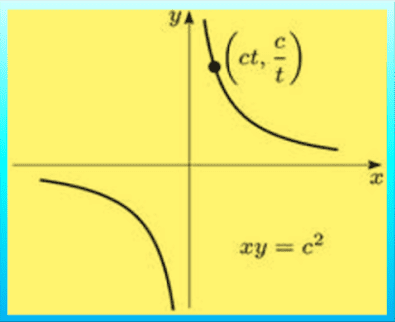• When the equation of the rect. hyperbola is xy = c2, then the asymptotes of it are the coordinate axes.
• The length of the latus rectum of rectangular hyperbola is the same as that of the length of transverse axis or conjugate axis.

### The rect. hyperbola with its asymptotes as the coordinate axes states the following properties and characteristics:

• The equation of the asymptotes of the rectangular hyperbola are: x = 0 and y = 0.
• The equation of the rect. hyperbola is xy = c2 and with the parametric representation as: x = ct and y = c/t; where, t ∈ R – {0}. So, the parametric point is (ct, c/t).
• The equation of the transverse axis of the rectangular hyperbola is: y = x.
• The equation of the conjugate axis of the rectangular hyperbola is: y = -x.
• The center of the rectangular hyperbola is at (0, 0).
• The vertices of the rectangular hyperbola are (c, c) and (-c, -c).
• The foci of the rectangular hyperbola are (√2c, √2c) and (-√2c, -√2c).
• The equations of the directrices of the rect. hyperbola are: x + y = ± √2c.
• Since, the transverse axis and the conjugate axis are same.Therefore, the length of latus rectum = 2√2c = length of T.A. = length of C.A
• The equation of the chord to the rect. hyperbola whose mid-point is given as (p, q) is: qx + py = 2pq.
• The equation of the tangent to the rect. hyperbola at a point P(x1, y1) is: x/x1 + y/y1 = 2.
• And, at parametric point P(t) i.e. P(ct, c/t), the equation of the tangent is: x/t + ty = 2c.
• The equation of the normal to the rect. hyperbola is: y – c/t = t2(x – ct).
• The equation of the chord to the rect. hyperbola, joining the points P(t1) and Q(t2) is written as: x + (t1t2)y = c(t1 + t2) and its slope, m = -1/t1t2.
• A rect. hyperbola which circumscribes a triangle, passes through the orthocentre of the triangle.
• If a circle intersects a rect. hyperbola at four points, then the mean value of the points of intersection is the mid-point of the line joining the centres of both, i.e. the circle and the hyperbola.

## Solved Example on Rectangular Hyperbola

Example-1: Find out the vertices and the foci of a rectangular hyperbola whose equaton is given as xy = 1.

Solution:

Given that, xy = 1.

Clearly, the given equation of the rect. hyperbola is of the form xy = c.

So, the vertices of the rect. hyperbola [xy = c] are (c, c) and (-c, -c).

• (1, 1) and (-1, -1)

And, the foci of the rect. hyperbola [xy = c] are (√2c, √2c) and (-√2c, -√2c).

• (√2, √2) and (-√2, -√2)

Answer: The vertices of the rect. hyperbola are (1, 1) and (-1, -1) and the foci of the rect. hyperbola are (√2, √2) and (-√2, -√2).

## Picked For You

Furthermore, these are some topics which might interest you: